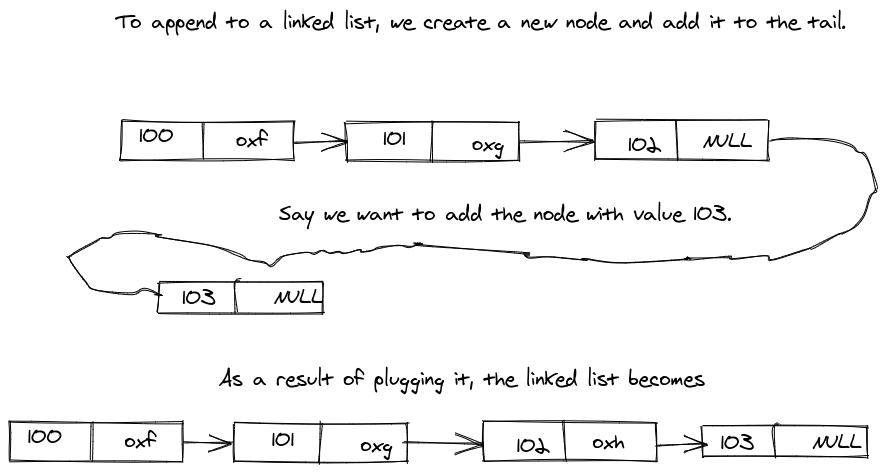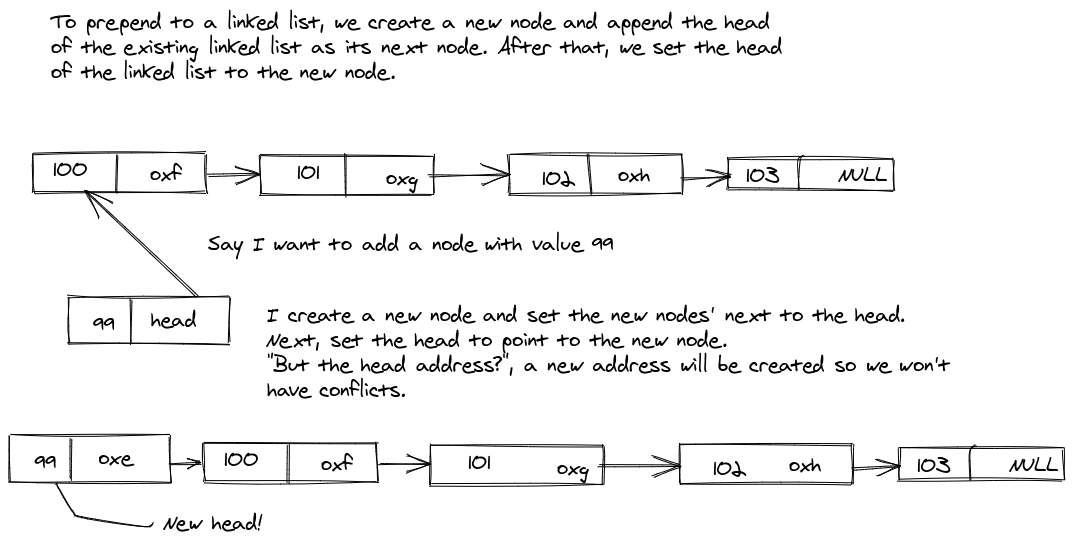In the previous post, I did an introduction to Linked Lists. The feedbacks and corrections were the motivation to continue, I'm happy you people found it nice, thanks!

Today, I'll be taking a look at the linked list class, its use and some methods. If you haven't read the introductory post to linked list, you should read it first.

Before you proceed, I am more comfortable writing in Python, I'd try my best to translate code to JavaScript and the likes :)

As I discussed in the previous post, a linked list is a continuous list of nodes. The nodes make the linked list, actually. The linked list class serves as a wrapper for adding functionalities and efficiently using the nodes. Take for instance, I want to append a node to the middle of a long chain of nodes, I'd have to perform it manually like this:

Node.py
``````nodes = Node(10)
nodes.next = Node(11)
nodes.next.next = Node(13)
nodes.next.next.next = Node(14)

# Insert a new node after 11.

new_node = Node(12)
new_node.next = nodes.next.next
nodes.next.next = new_node``````

Stressful, isn't it? Imagine I need to perform this same operation for a couple of nodes, say 10, I have to manually do this every time. This brings forth unnecessary code bulkiness and complexity - space. Having a Linked List class with a method solves this all.

The Linked List class is an independent class. The initialisation of nodes in the class uses the `Node` class still. Here is a linked list class implementation:

In Python:

``````import Node # You could as well fix the Node class here.

def __init__(self, value):

In JavaScript:

``````// You should export the Node class.

import Node from './Node'

constructor(value = 0){
}
}``````

1. `append` - This adds a new node after a head node or tail node
2. `prepend` - This adds a new node before the existing nodes in the linked list, making it the new head.

Let's start with the `append` method:

In Python

``````class LinkedList:
def __init__(self, value):

def append(self, value):
new_node = Node(value)

return

In JavaScript

``````class LinkedList {
constructor(value = 0){
}

append(value) {
let new_node = new Node(value)
}

}
}
}``````

I'll break down the process in the code block in a quick summary:

The first thing the method does is to create a new node and store it in a variable. Next, it checks if there's only one node in the list, if that turns out to be true, append the node to the head then return. However, if the head node isn't the only node in the linked list, traverse the node till we get to the tail and append it there.

Here's a diagramatic explanation:Let's implement the `prepend` method, this is actually very simple. Just after the `append` method, add the following block of code:

In Python

``````def prepend(self, value):
new_node = Node(value)

In JavaScript:

``````prepend(value) {
let new_node = new Node(value)
return current
}``````

The prepend method is self-explanatory. What it does is it appends the head of a linked list to a newly created node and change the head of the linked list to the newly created node.

Here's a diagramatic explanation of the prepend method:The last method I'll be implementing in this blogpost is the `printList` method. This method enables us output ( or print ) all the node values in the linked list as an array. Implement this method after the `prepend` method.

In Python:

``````def printList(self):
output = []
return output``````

In JavaScript:

``````printList() {
The `printList` method traverse each node in the linked list and stores the value in the `result` array.